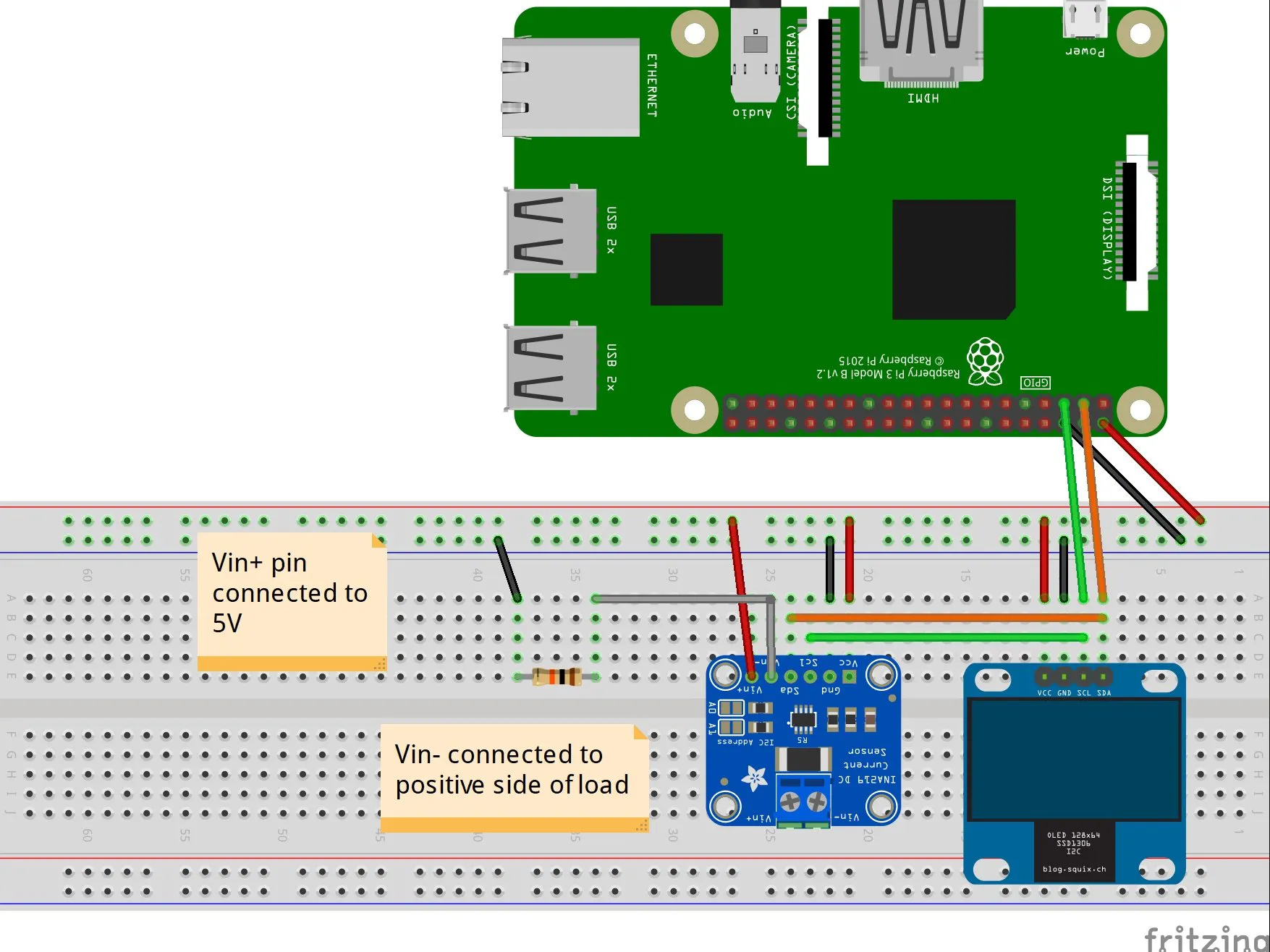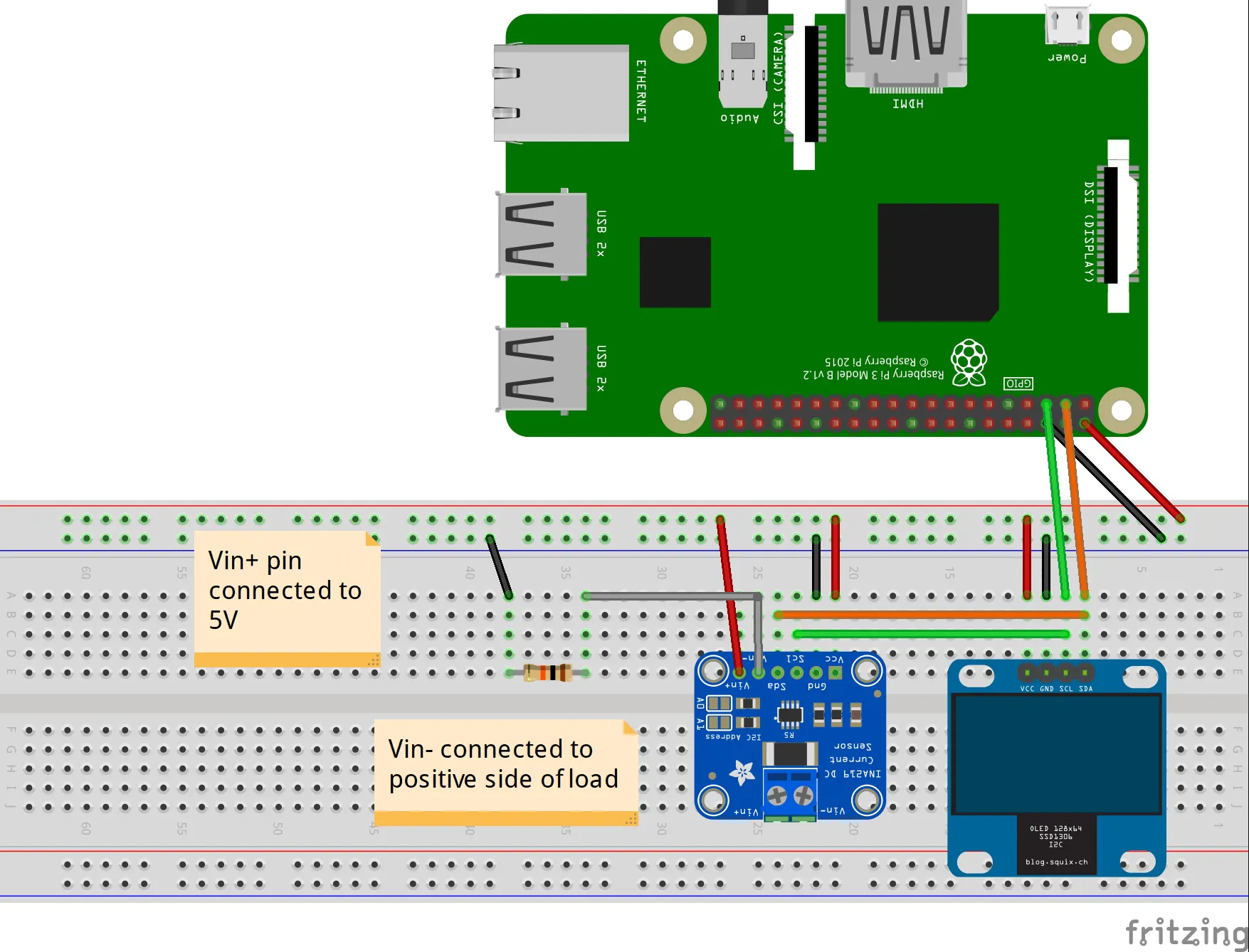Published

# DIY Power Logger Using I2C & Python

Make your own power logger for electronics that you use in the house using INA219 chip, SSD1306 OLED display, Raspberry Pi 3, and Python.

BeginnerFull instructions provided8 hours1,836## Things used in this project

### Hardware componentsRaspberry Pi 3 Model B
×1
×1
 Adafruit MONOCHROME 0.96" 128X64 OLED GRAPHIC DISPLAY
×1Jumper wires (generic)
×1Resistor 10k ohm
×1LED (generic)
×1

## Schematics

### Fritzing Sketch### 10K resistor setup

Data & graphs for 10K resistor

### LED setup

Data & graphs for LED setup

### FTDI setup

Data & graph for FTDI breakout board

## Code

### Source code

Python
Python source code file. First the OLED displaying is configured then in the while loop data is read periodically from the sensors & appended in the .xlsx file.
```import time

from ina219 import INA219
from ina219 import DeviceRangeError
from time import sleep

from PIL import Image
from PIL import ImageDraw
from PIL import ImageFont

from openpyxl import Workbook
from openpyxl.chart import LineChart, ScatterChart
from openpyxl.chart import Reference, Series

# INA219 shunt value & reset pin for SSD1306 display
SHUNT_OHMS = 0.1
RST = None

# 128x64 display with hardware I2C:
disp = SSD1306_128_64(rst=RST)

# Initializa libray
disp.begin()

# Clear display
disp.clear()
disp.display()

# Create blank image for drawing.
# Make sure to create image with mode '1' for 1-bit color.
image = Image.new('1', (disp.width, disp.height))

# Get drawing object to draw on image.
draw = ImageDraw.Draw(image)

# Draw a black filled box to clear the image.
draw.rectangle((0,0,disp.width,disp.height), outline=0, fill=0)

# Padding for text aligment. This can be used or not
x = 0

# Configure INA219 sensor
ina = INA219(SHUNT_OHMS)
ina.configure()

# Load Minecraftia-Regular.ttf font file (should be in the same folder
# with the script
font = ImageFont.truetype('Minecraftia-Regular.ttf', 8)
print("Press Ctrl+C to quit.")
sleep(3)

# Create Workbook
wb = Workbook()
ws = wb.active
ws.title =  'Sensor Output'
ws['A1'] = 'Bus Voltage (V)'
ws['B1'] = 'Bus current (mA)'
ws['C1'] = 'Power (mW)'
ws['D1'] = 'Shunt Voltage (mV)'

try:
while True:
# Draw a black filled box to clear the image.
draw.rectangle((0,0,disp.width,disp.height), outline=0, fill=0)

# Round floating
bus_V = round(ina.voltage(), 5)
bus_I = round(ina.current(), 5)
power = round(ina.power(), 5)
shunt_V = round(ina.shunt_voltage(), 5)
load_V  = round((bus_V + (shunt_V/1000)), 5)

print("bus voltage: %s V" % bus_V)
print("bus current: %s mA" % bus_I)
print("power: %s mW" % power)
print("shunt voltage: %s mV" % shunt_V)

# Draw information on the OLED screen
draw.text((x, top), "INA219 Current Sensor", font=font, fill=255)
draw.text((x, top+8),  "#####################", font=font, fill=255)
draw.text((x, top+16), "Bus V: " + str(bus_V) + " V", font=font, fill=255)
draw.text((x, top+24), "Bus Curr: " + str(bus_I) + " mA", font=font, fill=255)
draw.text((x, top+32), "Power: " + str(power) + " mW", font=font, fill=255)
draw.text((x, top+40), "Shunt V: " + str(shunt_V) + " mV" , font=font, fill=255)
draw.text((x, top+48), "Load V: " + str(load_V) + " V", font=font, fill=255)
draw.text((x, top+56), "#####################", font=font, fill=255)

disp.image(image)
disp.display()
sleep(.1)
# Append values to Workbook

except DeviceRangeError as e:
# Current out of device range with specified shunt resistor
print e

except KeyboardInterrupt as e:
print("\nProgram closed")

finally:
# Draw a black filled box to clear the image.
draw.rectangle((0,0,disp.width,disp.height), outline=0, fill=0)
disp.image(image)
disp.display()

# Create line chart for Load Voltage
c1 = LineChart()
c1.style = 13
c1.y_axis.title = 'V'
c1.x_axis.title = 'Row index'
data = Reference(ws, min_col=5, min_row=1, max_col=5, max_row=ws.max_row)

# Create line chart for Bus current
c2 = LineChart()
c2.title = "Bus current"
c2.style = 13
c2.y_axis.title = "mA"
c2.x_axis.title = "Row Index"
data = Reference(ws, min_col=2, min_row=1, max_col=2, max_row=ws.max_row)

# Create line chart for Power consumption
c3 = LineChart()
c3.title = "Power"
c3.style = 13
c3.y_axis.title = "mW"
c3.x_axis.title = "Row Index"
data = Reference(ws, min_col=3, min_row=1, max_col=3, max_row=ws.max_row)

# Save Workbook
wb.save('Power Logger_' + time.strftime('%d_%b_%Y_%H_%M_%S') +'.xlsx')
```

### Minecraft font file

Textile
This is the tff file loaded in the script. It has to be in the same folder with the script.
`No preview (download only).`

## Credits

### Aurelian Ioan

2 projects • 7 followers
Thanks to GreatScott!.